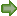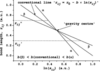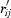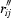STRUCTURAL SCIENCECRYSTAL ENGINEERINGMATERIALS
ISSN: 2052-5206
Volume 65| Part 3| June 2009| Pages 401-402

## On the correlations between the polyhedron eccentricity parameters and the bond-valence sums for the cations with one lone electron pair. Addendum

aDepartment of Chemistry and Research Institute for Physics and Chemistry of Solids, Uzhgorod National University, Pidgirna Street 46, Uzhgorod 88000, Ukraine
*Correspondence e-mail: vasylsidey@yahoo.com

(Received 21 January 2009; accepted 9 March 2009; online 2 May 2009)

Systematic variations of the bond-valence sums calculated from the poorly determined bond-valence parameters [Sidey (2008), Acta Cryst. B64, 515–518] have been illustrated using a simple graphical scheme.

Recent discussion on the reliability of the conventional bond-valence (BV) parameters, r0 and b, determined using the universal constant' b = 0.37 Å (Krivovichev & Brown, 2001; Locock & Burns, 2004; Sidey, 2008; Wang & Liebau, 2009) has stimulated writing of this addendum to the earlier communication of the author (Sidey, 2008). The main goal of this addendum is to explain, as simply as possible, the reason for the systematic variations of the bond-valence sums (BVSs) calculated from the poorly determined BV parameters (Sidey, 2008). All the terms, symbols and abbreviations used here correspond to those used in the aforementioned original communication of the author (Sidey, 2008).

The most commonly adopted empirical expression for the relationship between the bond valences sij and the bond lengths rij (Brown & Altermatt, 1985; Brese & O'Keeffe, 1991) can be rewritten as rij = r0b × ln(sij). Hence, every sijrij' curve can be uniquely represented as a straight line y = A + Bx by using the ln(sij) − rij' coordinate system (Fig. 1). The mathematical slope and the y intercept of the above straight lines are equal to −b and r0, respectively. The traditional calculation scheme for determining the conventional BV parameters (Brown & Altermatt, 1985; Brese & O'Keeffe, 1991) is based on the rather rough assumption that the mathematical slopes of all ln(sij) − rij' straight lines are equal to −0.37 Å; so that all observed ln(sij) − rij' correlations are approximated with the constant-slope (conventional) straight lines rij = r0 – 0.37ln(sij). If the real value of the b constant is not 0.37 Å, then the optimized' conventional straight line obtained for a given ion pair merely goes through the gravity centre' datapoint 〈ln(sij)〉 − 〈rij〉'1 of the whole observed ln(sij) − rij' dataset. As every well-determined coordination shell in the traditional calculation scheme is considered to be an independent observation' (Brown & Altermatt, 1985; Brese & O'Keeffe, 1991), the coordinates of the above gravity centre' datapoint observed for a given ion pair are usually close to the ln(sij) − rij' data corresponding to the coordination shells with the most frequently occurring (typical') coordination number(s).Figure 1 Typical relations between the straight lines rij = r0 − b × ln(sij) of different slopes (for simplicity, all the values are given in arbitrary units, a.u.).

Fig. 1shows the relations between the straight lines rij = r0b × ln(sij) of different slopes. The α and β straight lines represent the idealized hypothetical ln(sij) − rij' correlations with the same gravity centre' datapoint; the α line has b > b(conventional) and the β line has b < b(conventional). If the real ln(sij) − rij' correlation is represented by the α straight line, then the conventional straight line gives overestimated and underestimated ln(sij) (and sij)2 values for the shorter () and longer () bond lengths, respectively. If the real ln(sij) − rij' correlation is represented by the β straight line, then the conventional straight line gives, in contrast, underestimated and overestimated ln(sij) (and sij) values for the shorter () and longer () bond lengths, respectively. The two different types of the systematic variations of the sij values observed for the α and β straight lines can be respectively denoted as the α and β variations.

Using the scheme presented here (Fig. 1), one can easily check the quality of the BV parameters reported for a given ion pair: if these BV parameters show systematic variations of the sij (and BVS) values within the short-bond and/or long-bond range(s), then the real sijrij' correlation is approximated poorly. Detecting the type of systematic variations observed for a given ion pair (α, β) can help find the actual value of the b parameter. It is important to note that overestimating and underestimating the sij values calculated for short and long (or vice versa) bonds belonging to the same irregular coordination shells can compensate for each other, so that the BVS values obtained for these shells can be quite plausible'; therefore, only regular (or nearly regular) shells should be used for checking the quality of the BV parameters.

The performance of the conventional BV parameters reported for a given ion pair is critically dependent: (a) on the real slope of the ln(sij) – rij' correlation and (b) on the range of the observed bond lengths rij. If the real mathematical slope is close to −0.37 Å and if the range of observed bond lengths is narrow, then the divergence between the real and conventional straight lines rij = r0b × ln(sij) is insignificant and, therefore, the conventional BV parameters reported for a given ion pair can be used with reasonable success. Otherwise, these BV parameters can be used successfully only within the rij range near to the above gravity centre' datapoint (Fig. 1),3 and must be revised. Since the coordinates of the gravity centre' datapoint observed for a given ion pair, 〈ln(sij)〉 and 〈rij〉, are usually close to the ln(sij) − rij' data corresponding to the typical' coordination shells (see above), even poorly determined conventional BV parameters can give reasonable BVS values in most cases! However, high-quality BV parameters should give reasonable BVS values not only for typical' coordination shells, but also for less-common shells formed by a given ion pair in ordered, stoichiometric and stable structures determined accurately and investigated under ambient conditions. Unfortunately, this requirement was ignored for a long time and a few theories were developed based on the axiomatic use of the universal constant' b = 0.37 Å (e.g. O'Keeffe & Brese, 1991; Wang & Liebau, 2007). In order to be properly validated, any BVM related theory (including that of Wang & Liebau, 2007) ought to operate with the BV parameters of the highest possible quality. Most of the conventional BV parameters used by Wang & Liebau (2007) in their theory show the aforementioned systematic α variations of the BVS values (Sidey, 2008) and, therefore, clearly must be revised.

### Footnotes

1〈ln(sij)〉 and 〈rij〉 denote the mean values for ln(sij) and rij.

2If ln(a) < ln(b), then a < b.

3Within a small section near to the aforementioned gravity centre' datapoint, every observed ln(sij) − rij' correlation can be considered as `well approximated'.

### ReferencesSTRUCTURAL SCIENCECRYSTAL ENGINEERINGMATERIALS